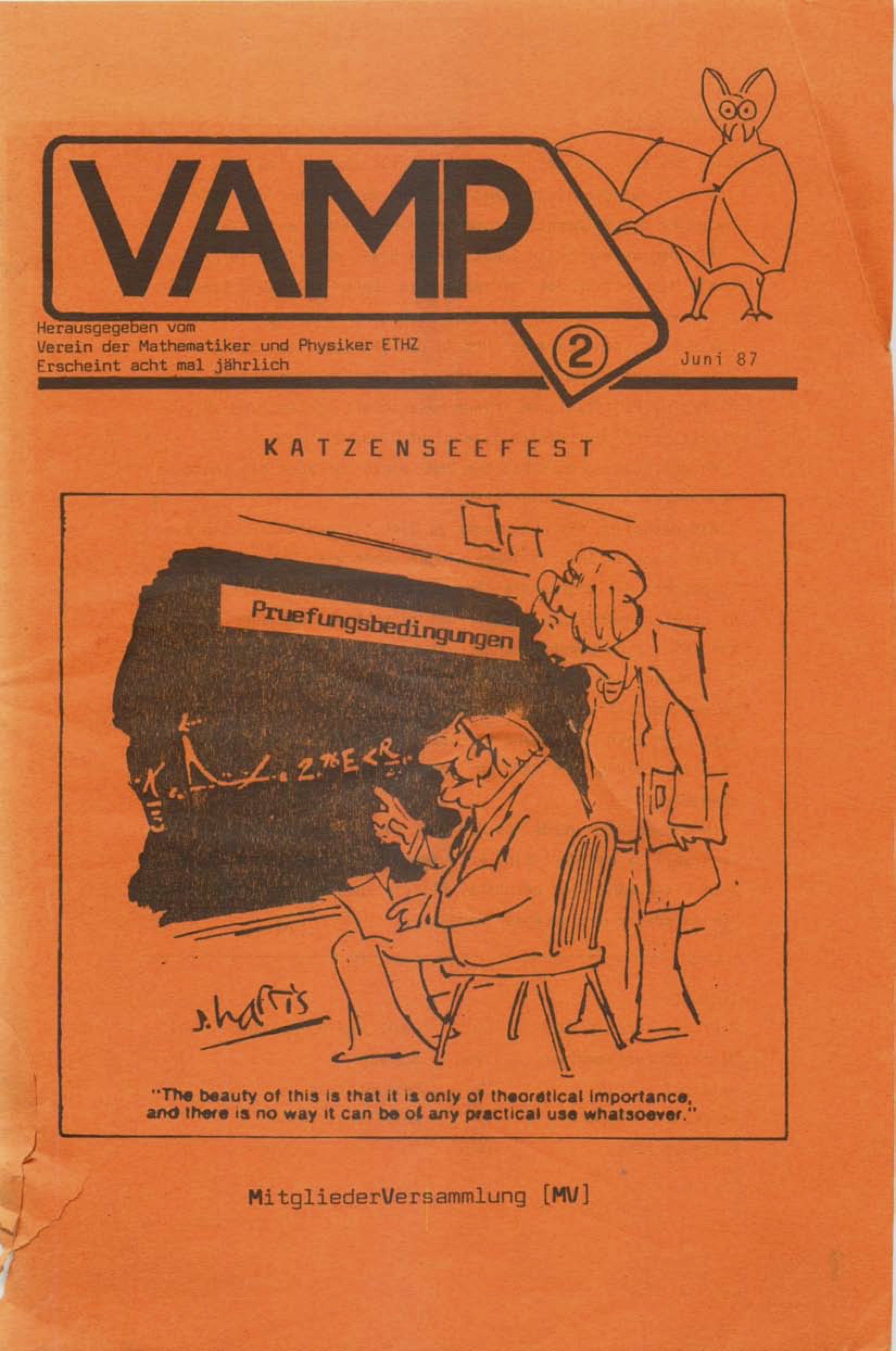# A damn hard riddle from 1987

Oliver Knill, posted 3/22/2019At ETH Zürich, we had the student organization VAMP (which stands for Verein Angehender Mathematiker und Physiker = student organization for future mathematicians and physisists). It published a journal like this edition from 1987 [PDF].
There is a riddle in the movie Fermat's room which reminded me about a contribution of mine [PDF] in that journal. I proved there a meta result which analyzes all possible puzzles of the type. Here is a concrete simple version of the problem:

 Given two integers x,y larger than 1. S knows the sum s=x+y but not the product p=xy and P knows the product p=x y but not the sum. Now there is the following dialog: P: ``I don't know x,y", S: ``I don't know x,y". P: ``I now know x,y". S: ``I now know x,y". What are the integers?

Try to solve the riddle first before scrolling down for the solution.
 TRY TO SOLVE THE PROBLEM FIRST!

 Solution: Because P does not know x,y, there are more than one possible factorizations. The smallest is 12 = 6*2 = 3*4. Their sum is 8 or 7. If the sum was 7, then S could figure out the numbers. So it is not 7. P knows now that the sum had to be 8 and so that x=6, y=2.

The harder question which is answered is: what possible dialogues can occur for which one can ask this riddle. This is what I tried to answer in that contribution. The most interesting riddle is the ``hard riddle":

 Given two integers x,y>1. S knows s=x+y but not p and P knows p=x y but not s. Now there is the following dialog: P: ``I don't know x,y". S: ``I don't know x,y". P: ``I don't know x,y". S: ``I don't know x,y". P: ``I now know x,y". S: ``I now know x,y". What are the integers?

Now the damn hard riddle:

 Prove that the above riddle is the hardest dialog riddle of that type.

I prove this by showing that otherwise, the dialog pattern will not end with a knowledge for both parties.

By the way, the riddle in Fermat's room is also of the type. It uses the fact that x+y+z with xyz=36 has no ambiguous solution if one knows that the largest of the x,y,z appears alone but that there are more than one solutions to xyz=36, x+y+z=a for any a. The problem is still hard if you see it the first time, but it is less surprising as we are confined to a finite set of possible factorizations. The above ``damn hard problem" does not give any framework and uses the intricate tension between sum and product (multiplicative and additive number theory).

 A student ask his teacher: how old are your 3 daughters? Teacher: ``if you multiply, you get 36. If you add, you get your house number." The student protests that it can not be solved. The teacher: ``You are right, the oldest plays the piano." How old are the daughters?

 Look at all the products. We have 6*6*1 = 36*1*1 = 18*2*1 = 6*2*3 = 4*3*3 = 9*2*2 with sums 13,38,21,11,10,13. The sum is ambiguous for 6*6*1 and 9*2*2. The last information gives 9,2,2.